2022-07-10
13分钟阅读时长

# 图解一致性模型

## 概述

### 解决什么问题？

• 容错性：即便分布式系统中几台机器不能工作，系统还能照常对外提供服务。
• 提升吞吐量：既然同一份数据存储在多个机器上，对该数据的请求（至少是读请求）能够分担到多个副本上，这样整个系统可以线性扩容增加更多的机器以应付请求量的增加。

In computer science, a consistency model specifies a contract between the programmer and a system, wherein the system guarantees that if the programmer follows the rules for operations on memory, memory will be consistent and the results of reading, writing, or updating memory will be predictable.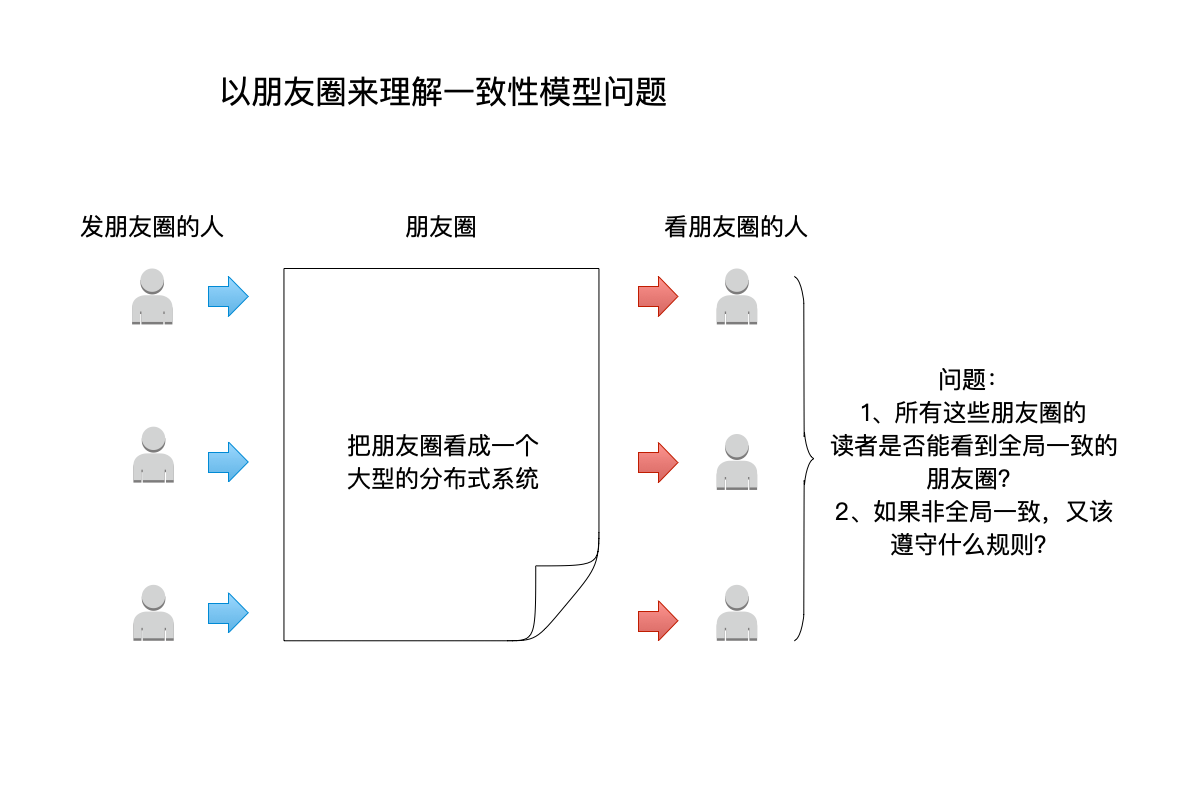• 不妨把朋友圈当成一个大型的分布式系统
• 这个分布式系统，对外提供了写入（发朋友圈）和读取（ 读朋友圈）的功能。
• 存储这些朋友圈数据的，肯定不止一台机器，因此这些机器在一起构成了这个大型的分布式系统。
• 不同的用户，发朋友圈的时候，也不一定都写入相同的一台机器。反之也是，在读朋友圈时也不一定会到同一台机器上读取数据。
• 朋友圈这个分布式系统，有两种客户端：发朋友圈的客户端负责写入数据，读朋友圈的客户端负责读取数据，当然很多时候同一个客户端既能读也能写。

• 这些看朋友圈的人，是否能看到全局一致的数据？即所有人看到的朋友圈都是同一个顺序排列的？

• 如果不同的人看到的朋友圈（包括评论）顺序各有不同，这些顺序又该遵守怎样的规则才是合理的？

### 一致性模型图例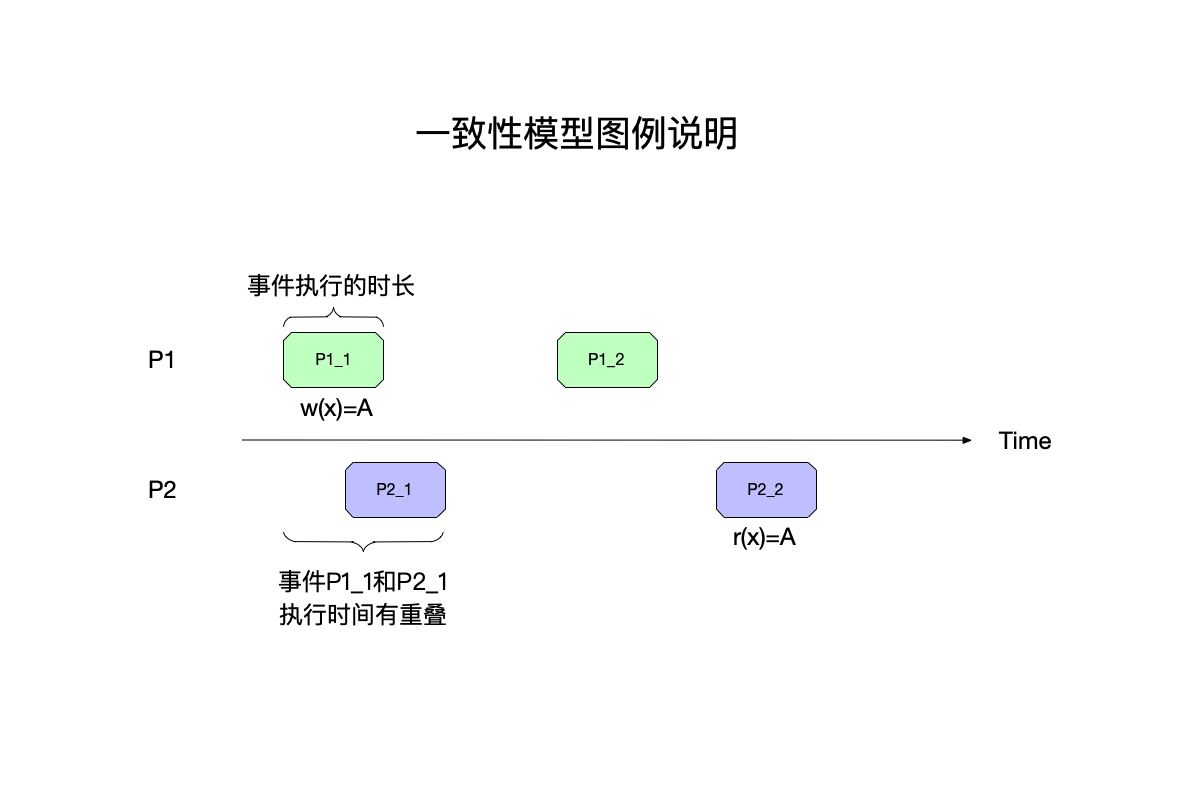• 最左边是分布式系统中的进程编号$P_1$、$P_2$。
• 横轴是时间轴，从左往右时间递增，但是这里并没有严格的时间刻度。
• 进程中发生的事件，事件的命名规则为进程编号_进程中的事件编号，比如$P_11$，除此以外：
• 事件有其开始、结束时间，使用一个矩形来表示一个事件的执行，这样矩形的宽度可以认为在时间轴上的宽度，即事件的执行时长。
• 事件与事件之间，在执行时间上可能发生重叠，如图中的$P_11$和$P_21$，这种有重叠的事件，被称为并发事件（concurrent event），在顺序上，并发事件之间可以认为谁先谁后都可以，后面会专门聊这部分。
• 使用不同的颜色来区分不同进程上发生的事件。
• 每个事件关联一个操作，为了简化问题目前只有读和写操作：
• w(x) = A：向变量x写入A。
• r(x) = A：从变量x读出A。

### 单进程下的事件排列

• 进程（也就是发表评论的人）是一个维度。
• 时间又是另一个维度，即这些评论出现的先后顺序。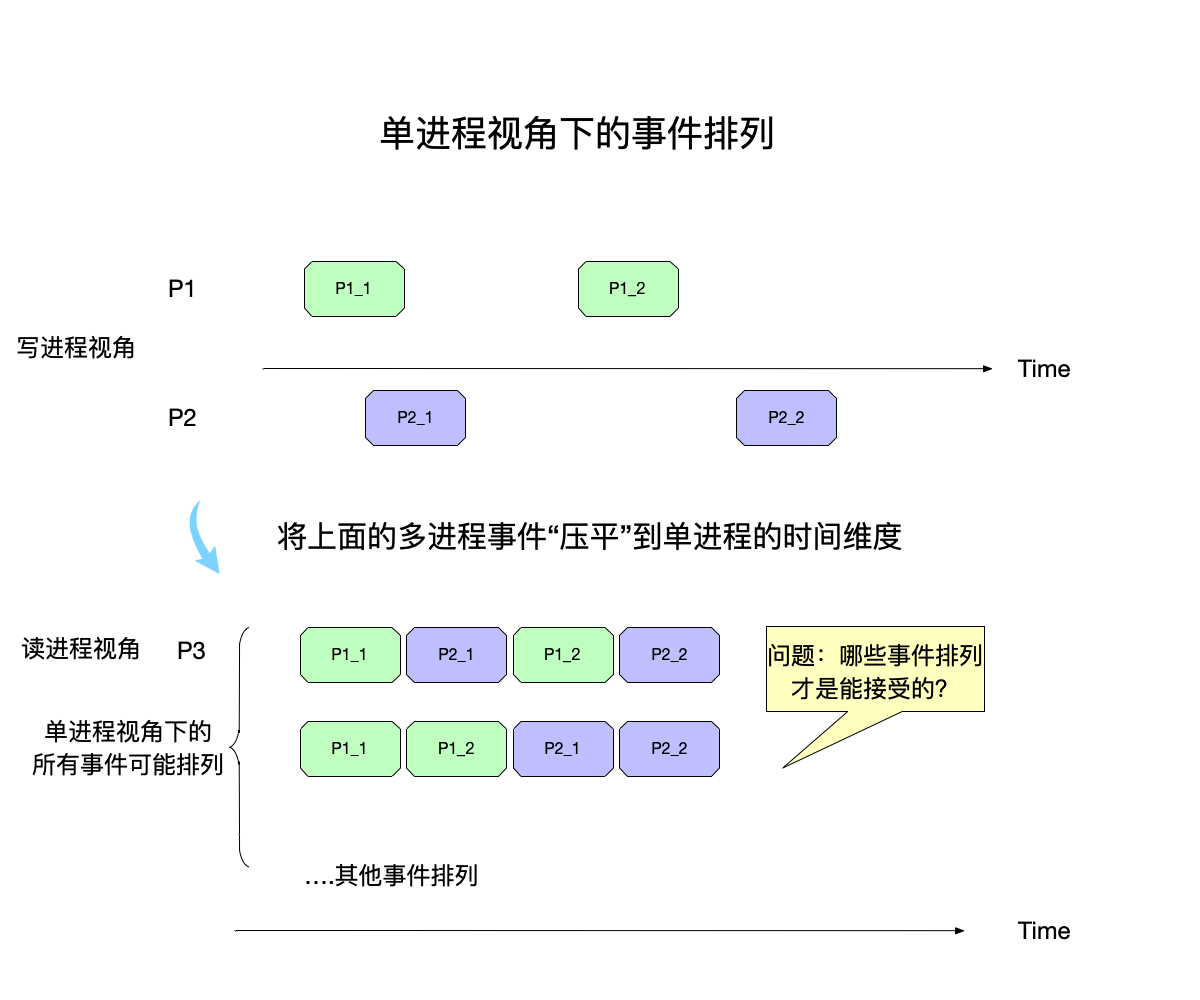{(a,b,c),(a,c,b),(b,a,c),(b,c,a),(c,a,b),(c,b,a)}


一致性模型就是要回答：在所有的这些可能存在的事件排列组合中，按照要求的一致性严格程度，哪些是可以接受的，哪些不可能出现？

## 一致性模型

### 顺序一致性

the result of any execution is the same as if the operations of all the processors were executed in some sequential order, and the operations of each individual processor appear in this sequence in the order specified by its program.

（任何执行的结果都与所有处理器的操作按某种顺序执行的情况相同，每个单独的处理器的操作按其程序指定的顺序出现在这个序列中。）

Requirement Rl: Each processor issues memory requests in the order specified by its program.

Requirement R2: Memory requests from all processors issued to an individual memory module are serviced from a single FIFO queue. Issuing a memory request consists of entering the request on this queue.

• 条件1：每个进程的执行顺序要和该进程的程序执行顺序保持一致。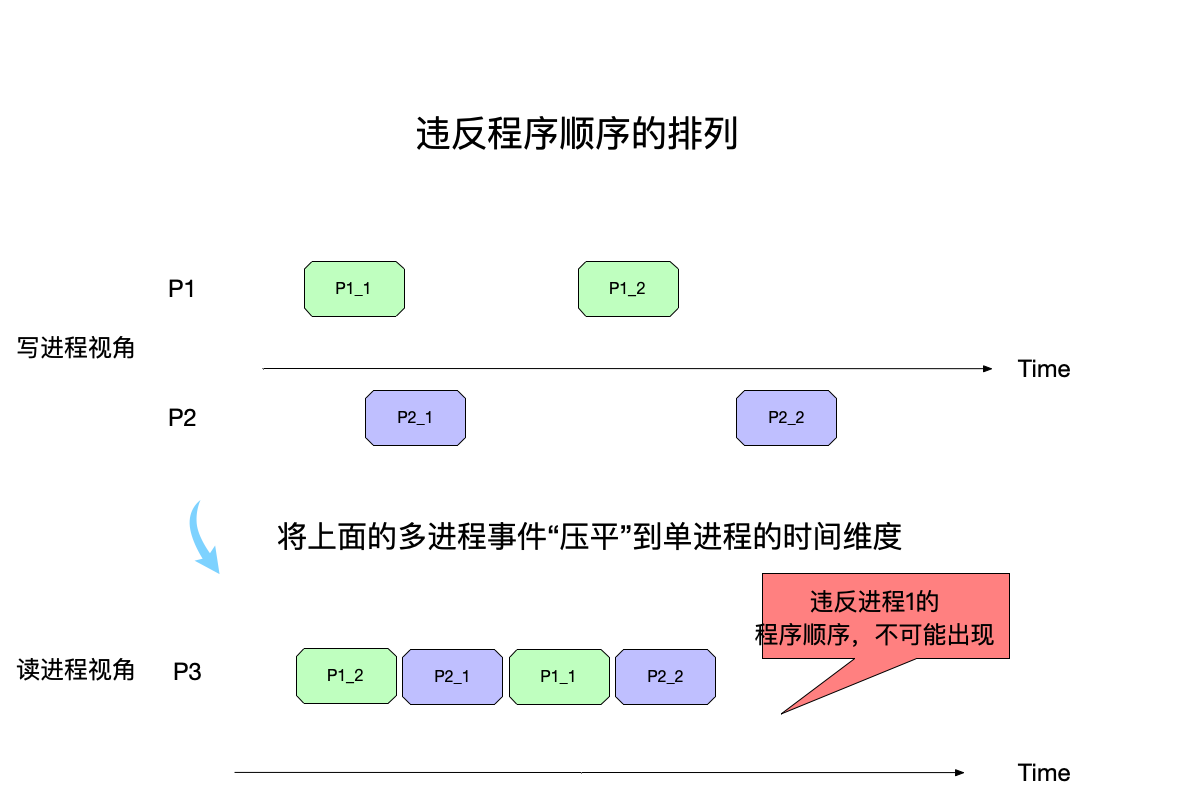• 进程${P_1}$视角下：程序的执行顺序是先执行 ${P_11}$，再执行 ${P_12}$。
• 但是到了P3视角下重排事件之后，${P_12}$出现在了事件${P_11}$前面，这是不允许出现的，因为违反了原进程${P1}$的程序顺序了。

• 条件2：对变量的读写要表现得像FIFO一样先入先出，即每次读到的都是最近写入的数据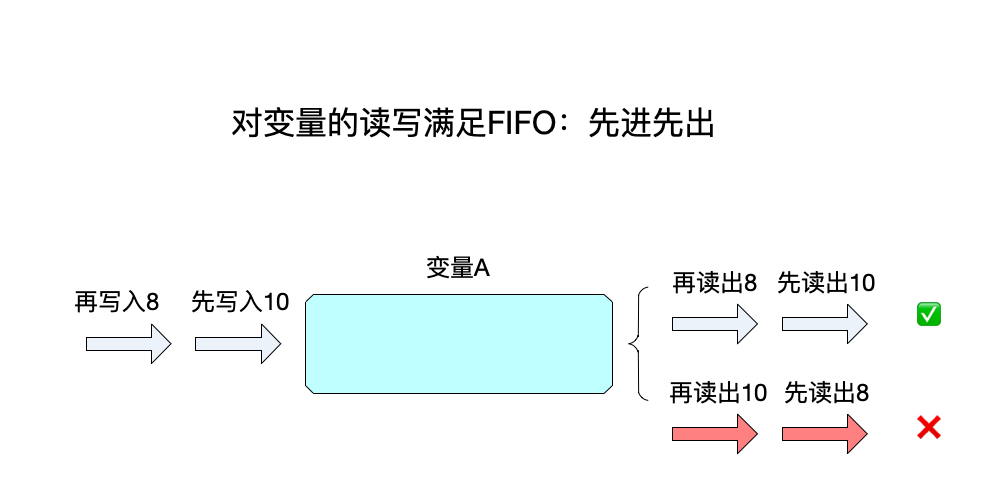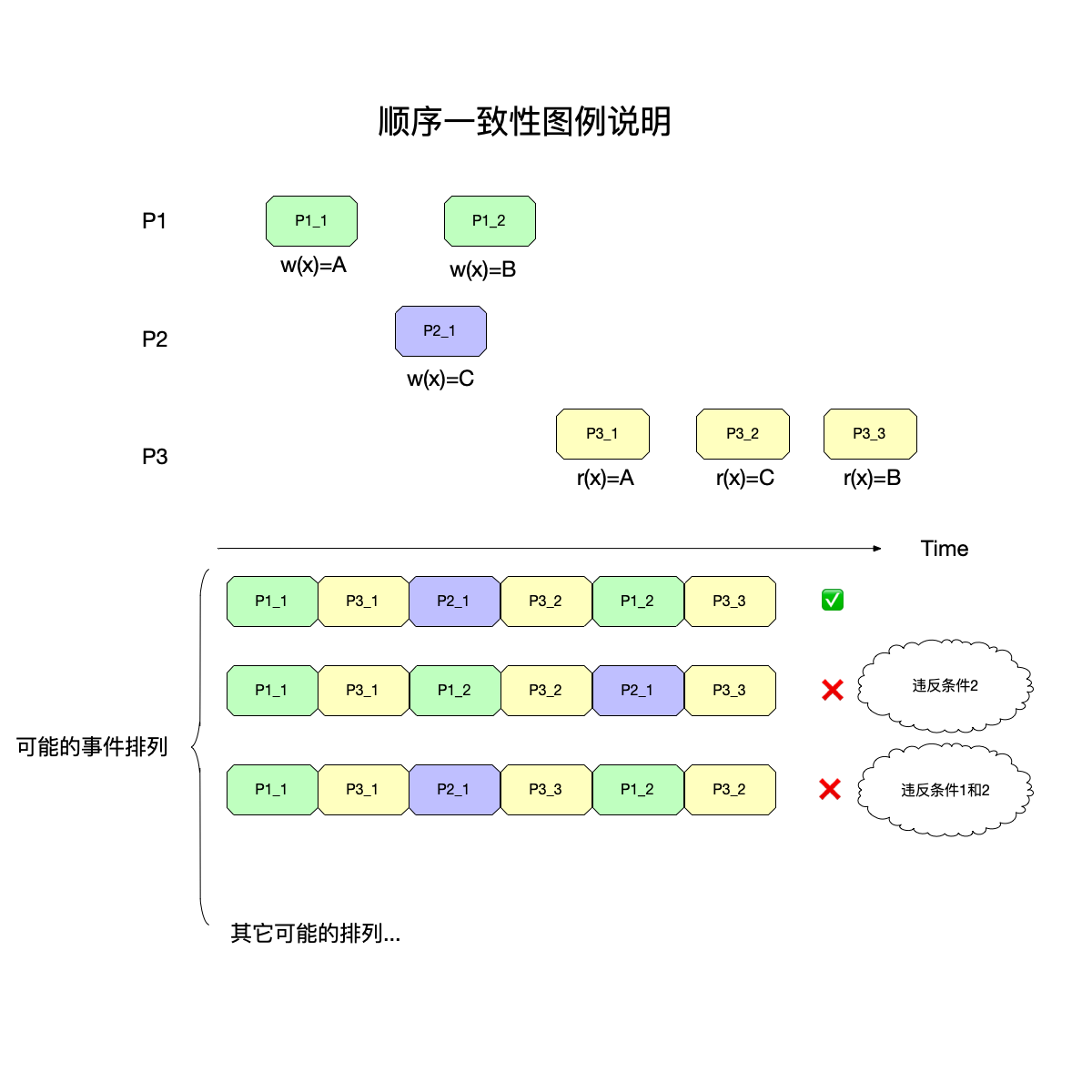• 进程${P_1}$：事件${P_11}$修改x为A，事件${P_12}$修改x为B。

• 进程${P_2}$：事件${P_21}$修改x为C。

• 进程${P_3}$：事件${P_31}$读出x为A，事件${P_32}$读出x为C，事件${P_31}$读出x为B。

• 第一种排列：
• 将事件对应的操作解读出来，那么执行顺序为：{w(x)=A,r(x)=A,w(x)=B,r(x)=B,w(x)=C,r(x)=C}。
• 可以看到，上面这个顺序，既没有违反条件1（因为同进程的程序顺序没有被打乱），也没有违反条件2（读出的都是开始写入的数据）。
• 第二种排列：
• 将事件对应的操作解读出来，那么执行顺序为：{w(x)=A,r(x)=A,w(x)=B,r(x)=C,w(x)=C,r(x)=B}。
• 由于${P_12}$和${P_21}$是并发事件，可以任意排列两者的顺序，这里选择先执行${P_12}$，可以看到：
• w(x)=B,r(x)=C，这违反了条件2。
• 第三种排列：
• 将事件对应的操作解读出来，那么执行顺序为：{w(x)=A,r(x)=A,w(x)=C,r(x)=B,w(x)=B,r(x)=C}。
• 这一次选择了先执行${P_21}$，可以看到：
• w(x)=C,r(x)=B以及w(x)=B,r(x)=C：违反了条件2。
• ${P_33}$先于${P_32}$执行，违反了条件1。

• 不能打乱单进程的程序顺序，同一个进程里的事件先后顺序要得到保留。
• 每次读出来的都是最新的值，而且一旦形成了一个排列，要求系统中所有进程都是同样的一个排列。

• 事件${P_31}$明明比事件${P_12}$和${P_21}$发生得更晚，但是只要在重排之后它是紧跟着${P_11}$的第一个读事件，就没有违反顺序一致性。在这个大前提下，事件${P_31}$甚至能出现在${P_12}$和${P_21}$之前，这看起来就很违反直觉了。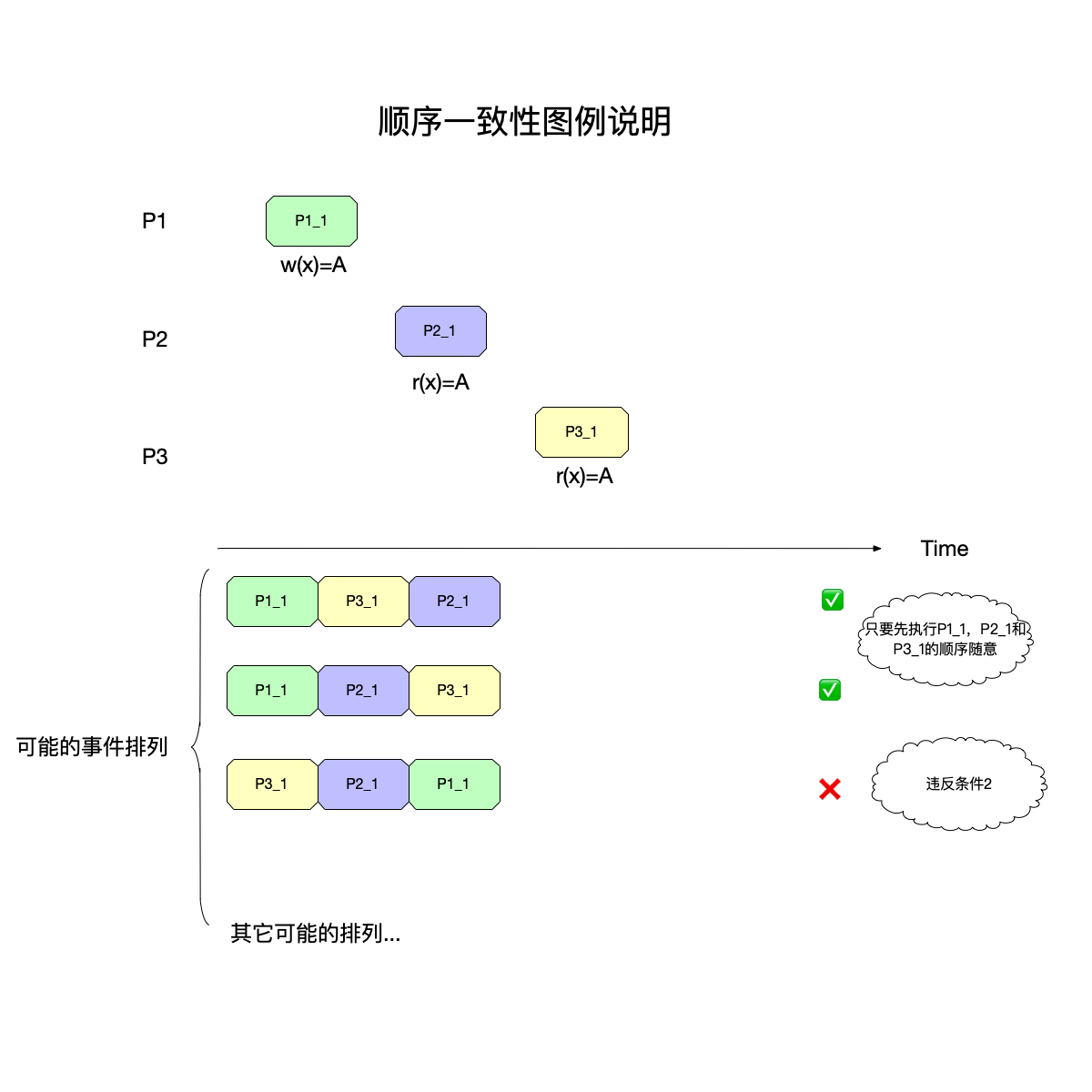• {${P_11}$,${P_21}$,${P_31}$}和{${P_11}$,${P_31}$,${P_21}$}都是对的，因为这两者都没有违反条件1和2。
• 只有最下面的{${P_31}$,${P_21}$,${P_11}$}才是错的，因为违反了条件2。

### 线性一致性

• 条件3：不同进程的事件，如果在时间上不重叠，即不是并发事件，那么要求这个先后顺序在重排之后保持一致。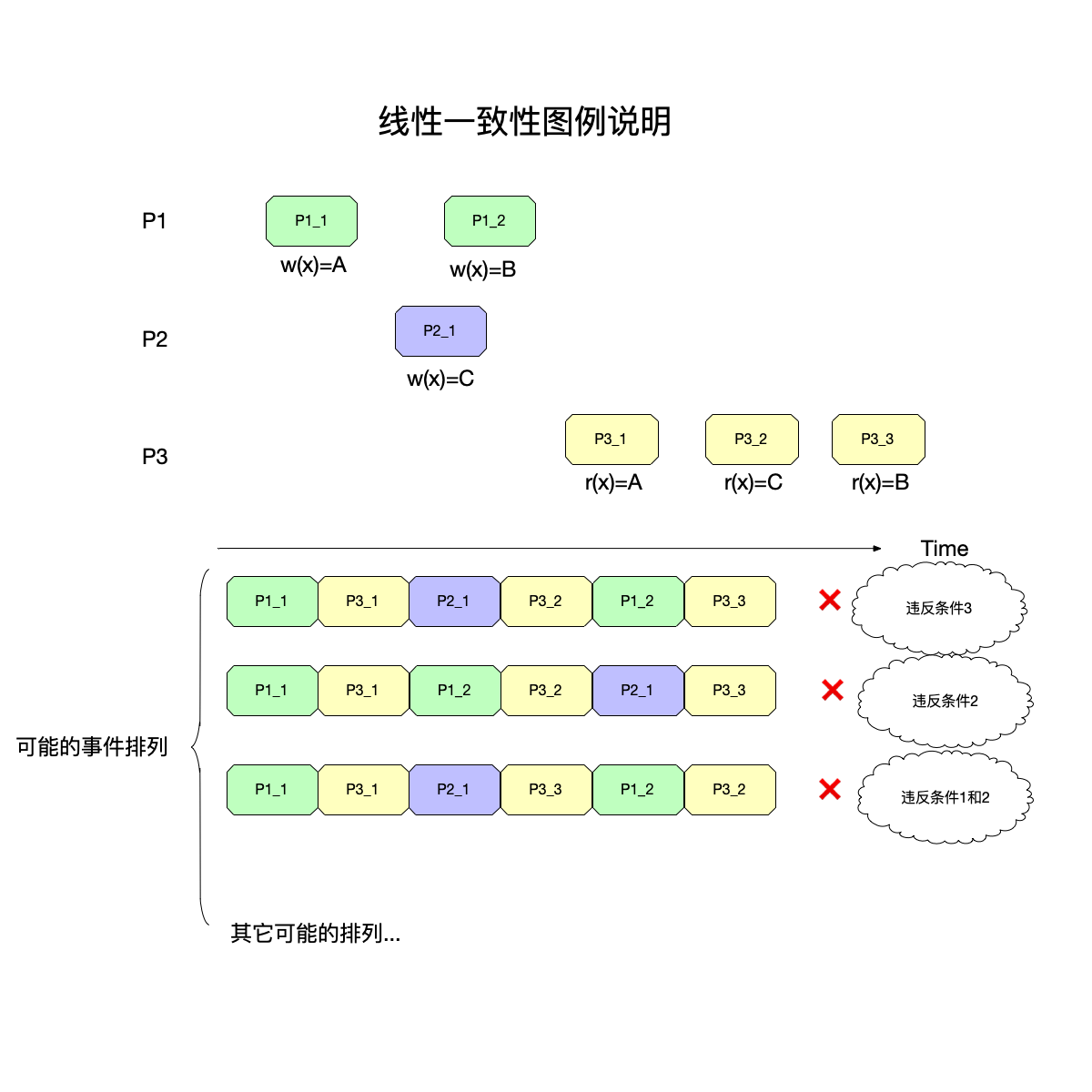• 事件${P_21}$和${P_12}$都在事件${P_11}$之后，这个顺序需要得到保持。
• 而事件${P_31}$在事件${P_21}$和${P_12}$之后，这个顺序也需要得到保持。
• 如果保持了前面两个顺序，那么${P_31}$执行的时候，必然读不出来A，而应该是B或者C（即${P_21}$或者${P_12}$的执行结果）。

### 因果一致性

• 引入符号$→$做为表示事件之间happen-before的记号。
• 在同一个进程中，如果事件$a$在事件$b$之前发生，那么$a → b$。（这是因为根据规则1，进程每次发出事件之后都会将本地的lamport时钟加一，于是可以在同一个进程内定义事件的先后顺序了）
• 在不同的进程中，如果事件$a$表示一个进程发出一个事件，事件$b$表示接收进程收到这个事件，那么也必然满足$a → b$。（这是因为根据规则2，接收进程在收到事件之后会取本地时钟和事件时钟的最大值并且+1，于是发出事件和接收事件尽管在不同的进程，但是也可以比较其lamport时钟知道其先后顺序了）
• 最后，happend-before关系是满足传递性的，即：如果$a → b$且$b → c$，那么也一定有$a → c$。

评论朋友圈这个行为来解释因果一致性再合适不过：

• 评论另一个用户的评论：相当于进程给另一个进程发消息，肯定要求满足happen-before关系，即先有了评论，才能针对这个评论发表评论。
• 同一个用户的评论：相当于同一个进程的事件，也是必须满足happen-before关系才行。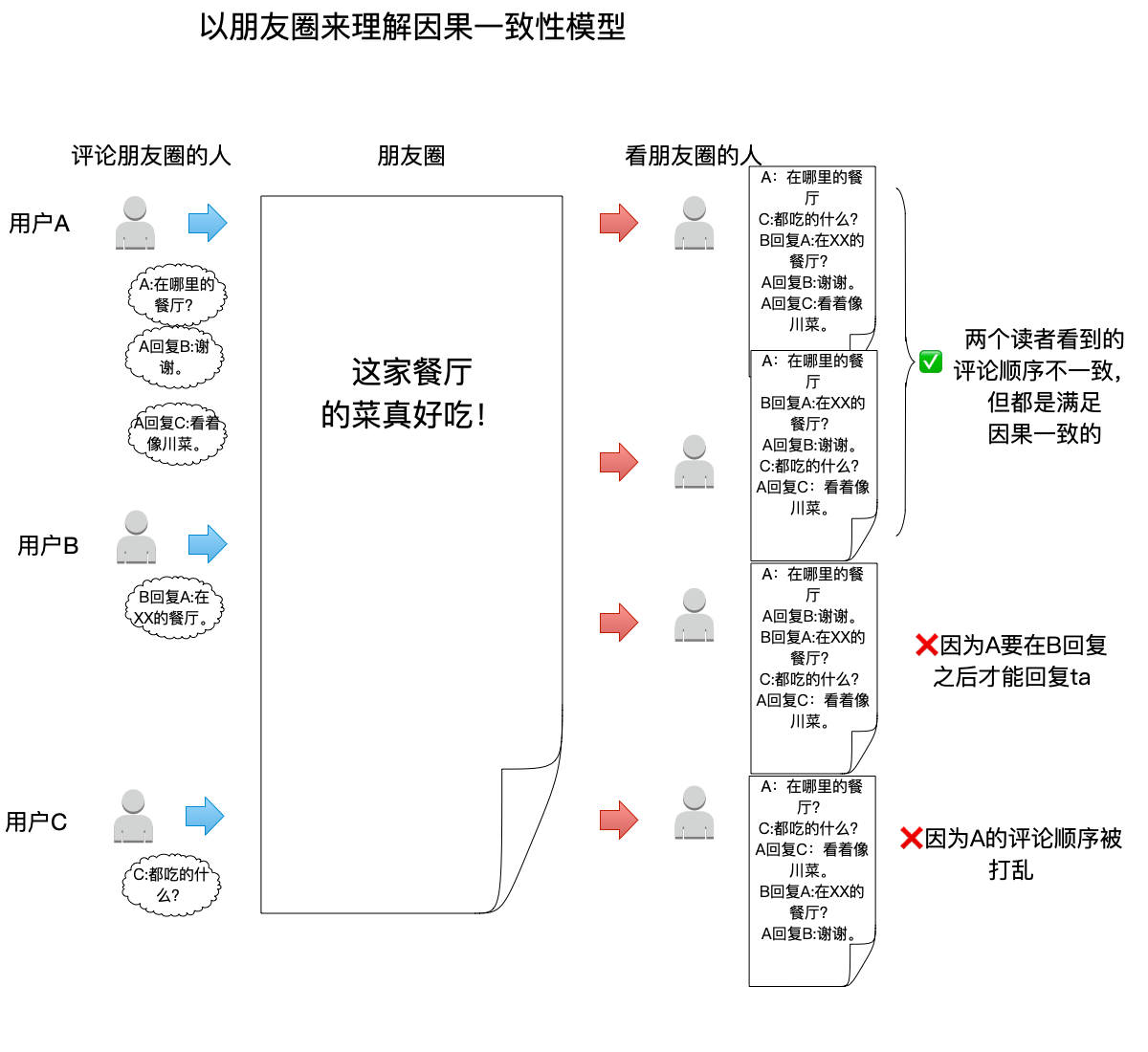• 最上面的两个读者，读到的顺序都满足因果一致性，因此即便是不一样的顺序也是正确的。
• 最下面的两个读者，顺序都不满足因果一致性：
• A回复B这个事件出现在了B回复A之前，不符合多进程之间的happen-before关系。
• A回复C这个事件在进程A中应该在A回复B之前，不符合同一进程的事件之间的先后顺序。

## 总结

• 在分布式系统中，多个进程组合在一起协调工作，产生多个事件，事件之间可以有多种排列的可能性。

• 一致性模型本质上要回答这个问题：按照该一致性模型定义，怎样的事件排列才符合要求？

• 顺序一致性和线性一致性都意图让系统中所有进程看起来有统一的全局事件顺序，但是两者的要求不同，顺序一致性：

• 条件1：每个进程的执行顺序要和该进程的程序执行顺序保持一致。
• 条件2：对变量的读写要表现得像FIFO一样先入先出，即每次读到的都是最近写入的数据。

只要满足这两个条件，顺序一致性对事件之间的先后顺序并没有硬性要求，而线性一致性在此基础上多了条件3：

• 条件3：不同进程的事件，如果在时间上不重叠，即不是并发事件，那么要求这个先后顺序在重排之后保持一致。
• 因果一致性是更弱的一致性，只要满足happen-before关系即可。由于happen-before关系实际上是由Lamport时钟定义的，这是一种逻辑时钟，所以不同的读者看到的先后顺序可能会有点反直觉，但是只要满足happen-before关系就是正确的。